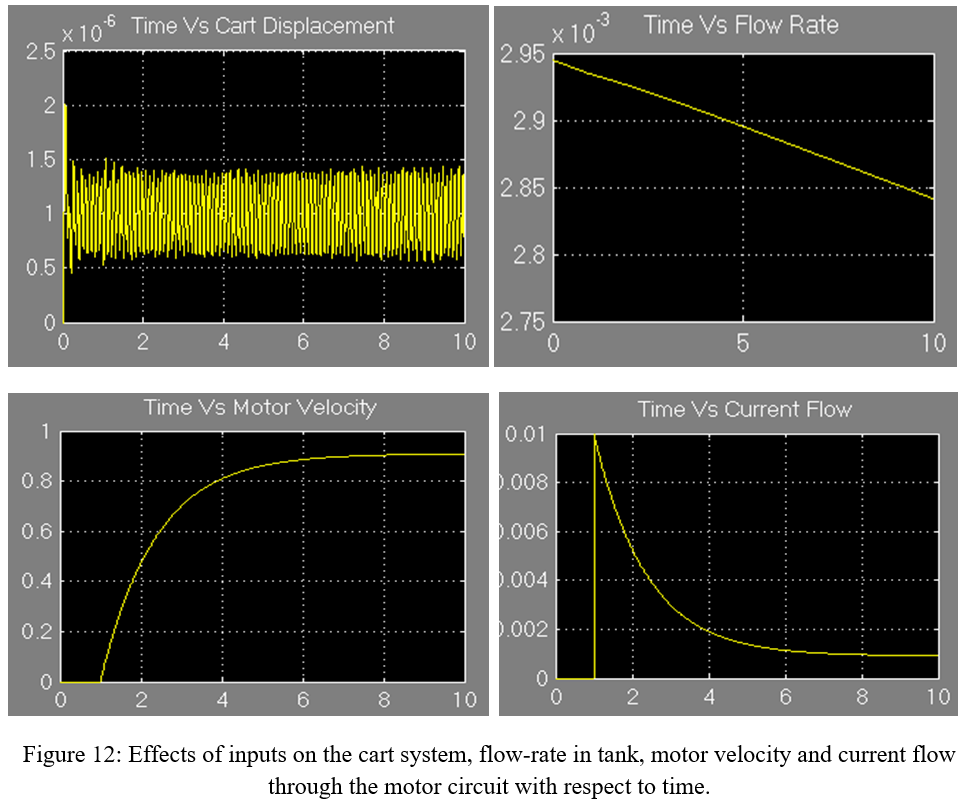### An Electro-mechanical System Model by MATLAB SIMULINK: Part 2

This is a continuation from Part-1

Observation 2: Step input (5 V) & Square Wave (5 V, 1 HZ)
In this case, both the step and square input source voltages are increased to 5 V. There is no significant changes in the cart displacement (figure 7). However, unstable condition is noticed for the response of the flow rate in the tank, motor angular velocity and current flow in the motor circuit. The flow in the tank oscillates and does not settle down. The motor angular velocity which was steady-state in the previous case now becomes unstable and oscillates (direction change). The same phenomenon is noticed for the current flow in the motor circuit as well. The possible explanation for these irregularities would be the non linearity of the complex coupled system where slight perturbation hampers the dynamic stability of the system.Observation 3: Step input (10 V) & Square Wave (10 V, 1 HZ)
Again the voltages for both of the inputs are changed to 10 V keeping the frequency of the square wave input same. From figure 8, the cart displacement is now totally in the negative side having a periodic waveform shape. The displacement is significantly less as it was observed before. The interesting point here is the drastic change of the responses of the flow rate, motor velocity and current flow in the circuit which are now behaving as linear system responses. From observation 1 and 2, it has already been realized from the shape of the cart displacement (complex periodic) that the system is inevitably non-linear. And, this is why, due to the change in the inputs, the dynamics of the system is irregular. It would be best to analyze the system more precisely (at least educated guess) if the eigenvalues of the differential equations are obtained.  However, simulation results also reflect some essential behaviour of the system.Observation 4: Step input (20 V) & Square Wave (20 V, 1 HZ)
The inputs are set to 10 V. From figure 9, cart displacement remains pretty much same as observation 3. However, the oscillations in the flow rate, motor velocity and current flow are observed again but with higher magnitudes in this case. As with the increase of both the input voltages, the system response becomes slower which eventually lengthen the overall simulation time.Observation 5: Step input (50 V) & Square Wave (50 V, 1 HZ)
Again both the input voltages are increased to 50 V. The cart displacement is similar to the previous observation. Also, from figure 10, the other three parameters show the oscillations as were in the last observation and similarly with higher magnitudes as well.Observation 6: Step input (1 V) & Square Wave (1 V, 5 HZ)
From observations 6 to 8, only the frequency of the square wave input will be increased keeping the voltages of both the inputs to 1 V. From figure 11, except the cart there is no oscillation in other components of the system. The motor reaches steady state after few seconds, the flow rate decreases almost linearly with time and the current flow decays exponentially. So, in this observation, only the cart oscillates with higher frequency.Observation 7: Step input (1 V) & Square Wave (1 V, 10 HZ)
Observation 7 is similar to the observation 6. The following figure suggests this close resemblance.Observation 8: Step input (1 V) & Square Wave (1 V, 30 HZ)
Here also, this observation is pretty much alike with the previous two observations. Figure 13 highlights the simulation results.#ElectroMechanical #MATLAB #SIMULINK #SystemDynamics #SquareWave #CartTankSystem #Blog #Blogger